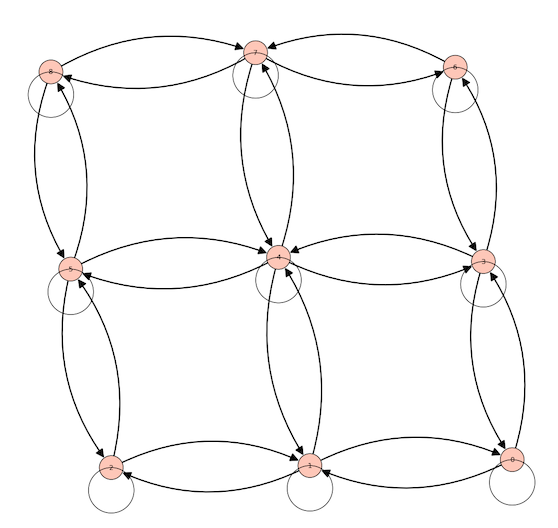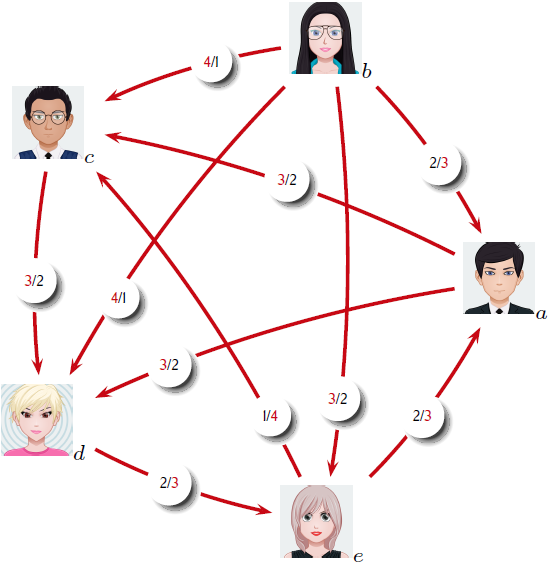# Graph plot: vertex attributes, node size, arrow head for loops, why are loops on nodes

Sorry, some of you think that I do not read the docs but the truth is that even reading them I cannot find my way.

Before using SageMath I was mainly working with PSTricks with which I have no problem drawing what I expect. If only it could be incorporated completely it would be a great moment.

Here is my problem: the code below works but I do not understand how to set the vertex attributes, the size of nodes, and so on.

A = matrix([
[1,1,0,1,0,0,0,0,0],
[1,1,1,0,1,0,0,0,0],
[0,1,1,0,0,1,0,0,0],
[1,0,0,1,1,0,1,0,0],
[0,1,0,1,1,1,0,1,0],
[0,0,1,0,1,1,0,0,1],
[0,0,0,1,0,0,1,1,0],
[0,0,0,0,1,0,1,1,1],
[0,0,0,0,0,1,0,1,1]])

D.show(figsize=16, vertex_size=100)But I do not understand how to set the vertex attributes, the size of the nodes, and so on. I even wonder why loops have no arrow head, why loops are plotted on top of nodes rather than underneath them. This is for my very strange since I have understood how to change the colors of the arcs. I truly need help (sorry to waste your time). I would also like to know if one can use images as vertex attributes.

vertex_attrs={'label':['1','2','3','4','5','6','7','8','9',]}


First of all my above code is only 3 lines. I have made many tries to insert vertex_attributes. None works.

Here is what I am able to achieve with PSTricks:I can provide the code. As I told you (this is also an answer to the comment to @dazedANDconfused), I work now mainly in html with SageCell because it's mandatory in these lockdown times. Most of my students (in economics) are poorly endowed in computers (some work with tablets or even phones). With html embedded SageCells I can overcome all those difficulties since computation are not realized in situ.

I was delighted in discovering Latex2js since their exemples are incredible. But all my trials are poor. It is not complete and their sandbox doesn't work (at least for me). But I think also that as SageMath is a pure killing software, it must be raised to that level.

Science is a pure public good. We are paid by government to increase common knowledge. But it is difficult to share it if you must pay for being published or to acquire software.

PSTricks code and node images as requested by slelievre

Note: my LaTeX preamble uses lots of PStricks packages and I do not remember which are used for this particular graph (I use also *graphix).

\begin{figure}[H]
\begin{center}
\begin{pspicture}(-3.2,-3.6)(3.6,3.6)
%\psgrid
%\pscircle(0,0){3cm}
\SpecialCoor
\rput(3;0){\rnode{A}{\includegraphics[scale=.25]{Avat0.png}$_a$}}
\rput(3;72){\rnode{B}{\includegraphics[scale=.25]{Avat1.png}$_b$}}
\rput(3;142){\rnode{C}{\includegraphics[scale=.25]{Avat2.png}$_c$}}
\rput(3;214){\rnode{D}{\includegraphics[scale=.25]{Avat5.png}$_d$}}
\rput(3;286){\rnode{E}{\includegraphics[scale=.25]{Avat4.png}$_e$}}
\ncarc[nodesep=3pt,linecolor=venetianred,linewidth=1.2pt]{->}{B}{A}
\ncarc[nodesep=3pt,linecolor=venetianred,linewidth=1.2pt]{<-}{C}{A}
\ncarc[nodesep=3pt,linecolor=venetianred,linewidth=1.2pt]{<-}{D}{A}
\ncarc[nodesep=3pt,linecolor=venetianred,linewidth=1.2pt]{<-}{A}{E}
\ncarc[nodesep=3pt,linecolor=venetianred,linewidth=1.2pt]{<-}{C}{B}
\ncarc[nodesep=3pt,linecolor=venetianred,linewidth=1.2pt]{<-}{D}{B}
\ncarc[nodesep=3pt,linecolor=venetianred,linewidth=1.2pt]{->}{B}{E}
\ncarc[nodesep=3pt,linecolor=venetianred,linewidth=1.2pt]{<-}{D}{C}
\ncarc[nodesep=3pt,linecolor=venetianred,linewidth=1.2pt]{<-}{E}{D}
\ncarc[nodesep=3pt,linecolor=venetianred,linewidth=1.2pt]{<-}{C}{E}
\NormalCoor
\end{pspicture}
\end{center}
\caption{\textsc{Scores dans les confrontations deux à deux}}\label{condorcetcomp}
\end{figure}


Node images: a · b · c · d · e.

Good luck working on that but I think it will be simpler to extend the show command to use PSTricks. That would make SageMath a pure wonder!

edit retag close merge delete

Can you share an example (maybe prepared with PSTricks) of what you would like to achieve?

Thank you for asking so many questions.

Thanks for often providing some code to get answerers something to start from.

It's not clear how you are using vertex_attrs above.

Regarding vertex size, try using vertex_size=400 or vertex_size=1600. Notice the difference?

If you're interested in producing professional looking graphs then LaTeX is the way to go using the packages tkz-graph and tkz-berge. These can work with Sage via the sagetex package. You can see some examples here or here or, for digraphs here. In these cases, the edges vertex sizes, labels, etc are set through LaTeX. The cooperation between Sage and LaTex is not "incorporated completely" but does a decent job.

dazedANDconfused it's a good suggestion. My problem is that i have decided to work mainly with html including sagecells because it's a trully wonderfull machinery. Many of my students haven't the capacity of a good computation because they are working on tablets even on phone. And we need this because right now in my university we are teaching on internet. With html embedded SageMAthCells this difficulty is overcomed (to the limit of what they use to navigate on internet). But until now there are some limitation I would appreciate to be adapted.

@Cyrille -- thanks for providing an example of desired output. Ideally, please also provide the PSTricks code, as well as the individual images for the nodes.